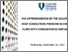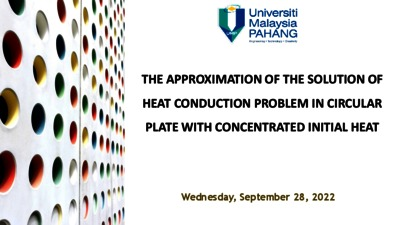# The approximation of the solution of heat conduction problem in circular plate with concentrated initial heat

Akhmedov, Abdulkasim and Salleh, Zuki and Rakhimov, Abdumalik (2022) The approximation of the solution of heat conduction problem in circular plate with concentrated initial heat. In: 3rd International Conference on Applied & Industrial Mathematics and Statistics 2022 (ICoAIMS 2022), 24-26 August 2022, Gambang. (Unpublished)Preview
PDF

## Abstract

Many problems of the engineering sciences can be solved using the modern methods of equations of mathematical physics, particularly elliptic differential equations play essential role in solving heat and mass transfer problems in engineering processes. In this paper, a numerical approximation of the solution of heat conduction problem in circular plate with initial concentrated heat is constructed by using the Riesz means of the spectral decompositions. Solution of heat transfer problems are subjected to the distributional boundary conditions and initial conditions.

Item Type: Conference or Workshop Item (Slide Presentation) Spectral Expansion; Heat Problems; Riesz means; Fourier Bessel series; Delta Function Q Science > QA Mathematics > QA300 Analysis Kulliyyah of Engineering > Department of Science Professor Abdumalik Rakhimov 04 Oct 2022 12:04 04 Oct 2022 12:04 http://irep.iium.edu.my/id/eprint/100302View Item